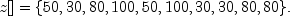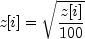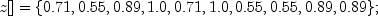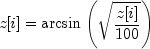JMSLTM Numerical Library 7.2.0
com.imsl.datamining.neural

Class UnsupervisedOrdinalFilter

• All Implemented Interfaces:
Serializable

public class UnsupervisedOrdinalFilter
extends Object
implements Serializable
Encodes ordinal data into percentages for input to a neural network. It also allows decoding, accepting a percentage and converting it into an ordinal value.

Class UnsupervisedOrdinalFilter is designed to either encode or decode ordinal variables. Encoding consists of transforming the ordinal classes into percentages, with each percentage being equal to the percentage of the data at or below this class.

Ordinal Encoding

In this case, x is input to the method encode and is filtered by converting each ordinal class value into a cumulative percentage.

For example, if x[]={2, 1, 3, 4, 2, 4, 1, 1, 3, 3} then nClasses=4, and encode returns the ordinal class designation with the cumulative percentages displayed in the following table. Cumulative percentages are equal to the percent of the data in this class or a lower class.

 Ordinal Class Frequency Cumulative Percentage 1 3 30% 2 2 50% 3 3 80% 4 2 100%

Classes in x must be numbered from 1 to nClasses.

The values returned from encoding or decoding depend upon the setting of transform. In this example, if the filter was constructed with transform = TRANSFORM_NONE, then the method encode will returnIf the filter was constructed with transform = TRANSFORM_SQRT, then the square root of these values is returned, i.e.,If the filter was constructed with transform = TRANSFORM_ASIN_SQRT, then the arcsin square root of these values is returned using the following calculation:Ordinal Decoding

Ordinal decoding takes a transformed cumulative proportion and converts it into an ordinal class value.

Example, Serialized Form
• Field Summary

Fields
Modifier and Type Field and Description
static int TRANSFORM_ASIN_SQRT
Flag to indicate the arcsine square root transform will be applied to the percentages.
static int TRANSFORM_NONE
Flag to indicate no transformation of percentages.
static int TRANSFORM_SQRT
Flag to indicate the square root transform will be applied to the percentages.
• Constructor Summary

Constructors
Constructor and Description
UnsupervisedOrdinalFilter(int nClasses, int transform)
Constructor for UnsupervisedOrdinalFilter.
• Method Summary

Methods
Modifier and Type Method and Description
int decode(double z)
Decodes an encoded ordinal variable.
int[] decode(double[] z)
Decodes an array of encoded ordinal values.
double encode(int x)
Encodes an ordinal category.
double[] encode(int[] x)
Encodes an array of ordinal categories into an array of transformed percentages.
int getNumberOfClasses()
Retrieves the number of categories associated with this ordinal variable.
double[] getPercentages()
Retrieves the cumulative percentages used for encoding and decoding.
int getTransform()
Retrieves the transform flag used for encoding and decoding.
void setPercentages(double[] percentages)
Set the untransformed cumulative percentages used during encoding and decoding.
• Field Detail

• TRANSFORM_ASIN_SQRT

public static final int TRANSFORM_ASIN_SQRT
Flag to indicate the arcsine square root transform will be applied to the percentages.
Constant Field Values
• TRANSFORM_NONE

public static final int TRANSFORM_NONE
Flag to indicate no transformation of percentages.
Constant Field Values
• TRANSFORM_SQRT

public static final int TRANSFORM_SQRT
Flag to indicate the square root transform will be applied to the percentages.
Constant Field Values
• Constructor Detail

• UnsupervisedOrdinalFilter

public UnsupervisedOrdinalFilter(int nClasses,
int transform)
Constructor for UnsupervisedOrdinalFilter.
Parameters:
nClasses - An int specifying the number of classes in the data to be filtered.
transform - An int specifying the transform to be applied to the percentages. Values for transform are: TRANSFORM_NONE, TRANSFORM_SQRT, TRANSFORM_ASIN_SQRT
• Method Detail

• decode

public int decode(double z)
Decodes an encoded ordinal variable.
Parameters:
z - A double containing the encoded value to be decoded.
Returns:
An int containing the ordinal category associated with y.
• decode

public int[] decode(double[] z)
Decodes an array of encoded ordinal values.
Parameters:
z - A double array containing the encoded ordinal data to be decoded.
Returns:
An int array containing the decoded ordinal classifications.
• encode

public double encode(int x)
Encodes an ordinal category.
Parameters:
x - An int containing the ordinal category. Must be an integer between 1 and nClasses.
Returns:
A double containing the encoded value, a transformed cumulative percentage.
• encode

public double[] encode(int[] x)
Encodes an array of ordinal categories into an array of transformed percentages.
Parameters:
x - An int array containing the categories for the ordinal variable. Categories must be numbered from 1 to nClasses.
Returns:
A double array of the transformed percentages.
• getNumberOfClasses

public int getNumberOfClasses()
Retrieves the number of categories associated with this ordinal variable.
Returns:
An int containing the number of categories associated with this ordinal variable.
• getPercentages

public double[] getPercentages()
Retrieves the cumulative percentages used for encoding and decoding. If a transform has been applied to the percentages then the transformed percentages are returned.
Returns:
A double array of length nClasses containing the cumulative transformed percentages associated with the ordinal categories.
• getTransform

public int getTransform()
Retrieves the transform flag used for encoding and decoding.
Returns:
An int containing the transform flag used for encoding and decoding.
• setPercentages

public void setPercentages(double[] percentages)
Set the untransformed cumulative percentages used during encoding and decoding. Setting percentages with this method bypasses calculating cumulative percentages based on the data being encoded. The percentages must be nondecreasing in the interval [0, 100], with the last element equal to 100. If this method is used it must be called prior to any calls to the encoding and decoding methods.
Parameters:
percentages - A double array of length nClasses containing the cumulative percentages to use during encoding and decoding.
JMSLTM Numerical Library 7.2.0